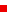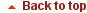search UMD     This SiteAndréTits, Dianne O'Leary receive new DoE optimization grantProfessor AndréTits (ECE/ISR) is the co-principal investigator for a new Department of Energy (DoE) grant, ?Interior-Point Algorithms for Optimization Problems with Many Constraints.? The Principal Investigator for this grant is Professor Dianne O?Leary (CS/UMIACS). The three-year, \$303,701 grant continues the research of an earlier grant in the same area.

About the research
The researchers will develop, analyze, and test algorithms for the solution of optimization problems with a very large number of inequality constraints, specifically, many more inequality constraints than variables. These types of problems arise, for example, in support vector machine training (for classification of data), network problems, and fine discretizations of semi-infinite programming problems such as those arising from partial differential equation models.

The researchers will consider problems related to linear programming (LP), convex or indefinite quadratic programming, and convex programming, as well as more general nonlinear programming.

Under the earlier grant, the PIs and their collaborators developed a framework for constraint reduction. For LP (i.e., minimization/maximization of a linear function subject to linear constraints), the idea is as follows. The solution of an LP is entirely determined by its objective function and a subset of the constraints, usually of size equal to the number of variables. Accordingly if the number of inequality constraints is much larger than the number of variables, then most of the constraints are, in a sense, irrelevant. Unfortunately, it is unknown at the outset which these are. This is what makes an LP hard to solve.

The framework already developed by AndréTits and Dianne O?Leary adaptively identifies, at each iteration of the solution process, a small set of seemingly critical constraints, and determines a search direction based on those constraints only. The algorithm was validated by theoretical analysis and numerical experimentation for linear and quadratic programming.

This new grant will allow the researchers to:

?Investigate approaches to efficiently deal with situations when the problem is ?sparse" (i.e., each variable is involved in only a small number of constraints);

?Improve the performance of algorithms to train support-vector machines, used for classifying data;

?Extend the work to general nonlinear programming (problems with nonlinear constraints); and

?Apply the techniques to entropy-based moment closure in gas dynamics.

Applications
As has been the case with previous algorithms and software developed by AndréTits and his coauthors (CFSQP, FFSQP, RFSQP and a number of Matlab scripts), the algorithms and software that will come out of the proposed research should have a significant impact in a wide range of application areas. This includes many areas of engineering (chemical, mechanical, electrical, transportation, controls...), but also areas such as medical research (epidemiology, oncology, radiology), statistics, finance and astronomy.

The work already completed under the earlier grant has had many connections to DoE labs; the new grant should produce algorithms and software of use to DoE as well as to the broader community. For example, algorithms to train SVMs could help detect anomalies in network interactions for homeland security, identify specific genome features, find supernovae, and estimate direction-of-arrival in signal processing.

 «Previous Story